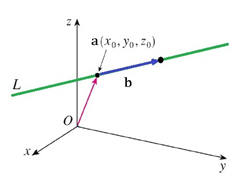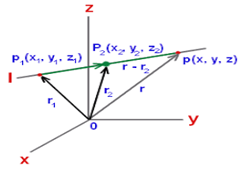#### A  symmetrical  form  of  the  line  of  intersection of the planesis Option 1)Option 2)Option 3)Option 4)As we learnt in

Cartesian eqution of a line -

The equation of a line passing through two pointsand parallel to vector having direction ratios asis given byThe equation of a line passing through two pointsis given by- whereinx-ay-b=0 and cy-z+d=0

Normal vector will beVector =So DRs are (a, 1, c)

Put y=1

x=a+b and z=c+d

So live becomesOption 1)This option is incorrect

Option 2)This option is correct

Option 3)This option is incorrect

Option 4)This option is incorrect

#### divya.saini